• ## Latex公式编号问题

万次阅读 多人点赞 2019-12-25 21:37:43
目录对某个公式编号/不编号 在写文章时，我们会遇到各种各样的对公式编号的要求，例如对某些公式标号而对另外一些公式不编号、对某些公式整体编号、对一个拆为几行的较长的公式的最后一行编号等。这篇文章总结了对...
目录对某个公式编号/不编号对某些公式整体编号较长公式拆行与编号
在写文章时，我们会遇到各种各样的对公式编号的要求，例如对某些公式标号而对另外一些公式不编号、对某些公式整体编号、对一个拆为几行的较长的公式的最后一行编号等。这篇文章总结了对上面三种情况的处理方法，后面遇到其他情况再回来补充。
对某个公式编号/不编号
latex中给我们提供了很多编辑公式的方法，具体可在终端（命令提示符窗口）输入如下命令查看官方文档，这里我们做简单总结。
texdoc lshort			%英文版
texdoc lshort-zh		%中文版
虽然latex提供了如下的方法用于输入单个公式，但是并不推荐使用这种方法。
$x^2+y^2=r^2$                   %行内公式
$$x^2+y^2=r^2$$                 %行间公式
单独成行的行间公式通常由equation环境包裹，equation环境自动为公式生成一个编号，这个编号可以通过 “\label” 和 “\ref” 生成交叉引用，还可以通过amsmath的 “\eqref” 命令为引用自动加上圆括号（若要使用 “\eqref” 命令，则须在导言区加上amsmath宏包），若引入了amsmath宏包，还可以使用 “\tag” 命令手动修改公式编号，或使用 “\notag” 命令取消为公式编号（若不想为公式编号，可直接使用equation*环境）。以下面的例子说明：
代码：
\documentclass{article}
\usepackage{amsmath}
\begin{document}
The Pythagorean theorem is:
\begin{equation}
a^2 + b^2 = c^2 \label{pythagorean}
\end{equation}
Equation \eqref{pythagorean} is
called ‘Gougu theorem’ in Chinese.
It’s wrong to say
\begin{equation}
1+1=3 \tag{dumb}
\end{equation}
or
\begin{equation}
1+1=4 \notag
\end{equation}
\end{document} 
编译结果如下图所示（因为存在交叉引用，故需要编译两次）：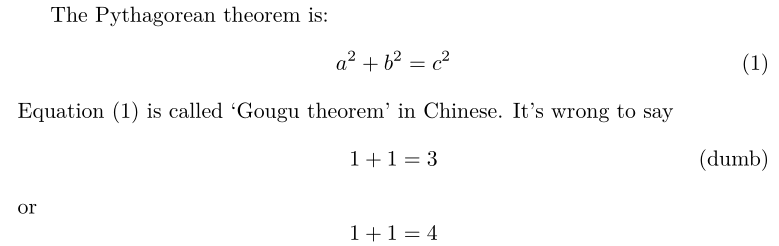注：

不允许有空行（分段）。行间公式也无法使用 ‘’\" 命令换行，排版多行公式的办法下面小节中给出。
所有的字母被当做数学公式中的变量处理，字母间距与文本模式不一致，也无法生成单词之间的空格。如果想在数学公式中输入正体的文本，可用amsmath提供的 “\text” 命令，举例如下：

\documentclass{article}
\usepackage{amsmath}
\begin{document}
$x^{2} \geq 0 \qquad \text{for all}\quad x\in R$
\end{document}
效果如下图所示：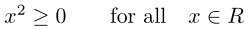对某些公式整体编号
某些时候要求我们对某几个公式整体进行编号，例如下面的例子：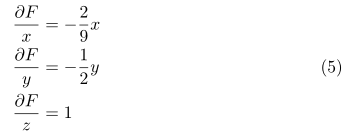方法一代码：
\begin{equation}
\begin{split}
\frac{\partial F}{x}&=-\frac{2}{9}x \\
\frac{\partial F}{y}&=-\frac{1}{2}y \\
\frac{\partial F}{z}&=1
\end{split}
\end{equation}
方法二代码（此方法依赖amsmath宏包）：
\begin{equation}
\begin{aligned}
\frac{\partial F}{x}&=-\frac{2}{9}x \\
\frac{\partial F}{y}&=-\frac{1}{2}y \\
\frac{\partial F}{z}&=1
\end{aligned}
\end{equation}
如果我们不需要等号对齐，而是居中对齐即可，那么用上述代码默认是又对其，下面的代码可使多行公式居中对齐。
\begin{gather}
\frac{\partial F}{x}=-\frac{2}{9}x \\
\frac{\partial F}{y}=-\frac{1}{2}y \\
\frac{\partial F}{z}=1
\end{gather}

较长公式拆行与编号
当我们遇到较长的公式，无法排版在一行时，就要求我们在适当的位置对公式进行拆分，并依照某种方式对齐，这里最常用的是align环境，它将公式用 “&” 隔为两部分并对齐，分隔符通常放在等号左边。align环境会给每行公式都编号，可使用 “\notag” 命令去掉某行编号，下面我们给出几个例子。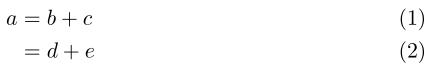代码：
\begin{align}
a&=b+c \\
&=d+e
\end{align}
若要取消对其中某行公式编号，则只需在相应的行使用 “\notag” 命令即可，如下：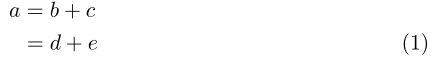代码：
\begin{align}
a&=b+c \notag \\
&=d+e
\end{align}
按加号对齐（为了对齐加号，我们将分隔符放在等号右边，这时需要给等号后添加一对括号 " {}"  以产生正常的）间距：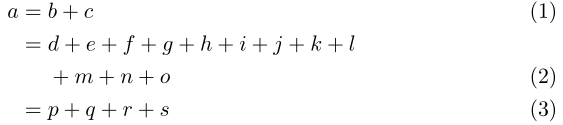代码：
\begin{align}
a ={} & b + c \\
={} & d + e + f + g + h + i
+ j + k + l \notag \\
& + m + n + o \\
={} & p + q + r + s
\end{align}


展开全文latex
• CSDN Markdown 公式编号 \tag{*} 标签设置公式的编号，公式编号右对齐。 \tag{*} 为手动编号。 While in box-free detectors, detection is performed merely on the set of feature maps $\Phi_{det}$, that is, $... CSDN Markdown 公式编号 \tag{*} 标签设置公式的编号，公式编号右对齐。 \tag{*} 为手动编号。 While in box-free detectors, detection is performed merely on the set of feature maps$\Phi_{det}, that is, $$Dets = \mathcal{H(\Phi_{det})} \tag{3}$$  While in box-free detectors, detection is performed merely on the set of feature maps $\Phi_{det}$, that is, $Dets = \mathcal{H(\Phi_{det})} \tag{3}$  展开全文• 1 解决同一章内自动编号不按顺序问题 选中不按顺序编号的第一个公式，点击MathType标签下的章&节，然后点击修改分隔符，在弹出的... 点击一次如果公式编号没有变化，重复上述操作，直到公式按顺序编号为止。 ... 1 解决同一章内自动编号不按顺序问题 选中不按顺序编号的第一个公式，点击MathType标签下的章&节，然后点击修改分隔符，在弹出的Modify Chapter/Section Break窗口中点Delete。 点击一次如果公式编号没有变化，重复上述操作，直到公式按顺序编号为止。展开全文深度学习 • ## Latex 子公式编号 千次阅读 2020-08-14 13:46:36 公式编号，并且后面要对整个公式交叉引用的办法： 多个子公式逐行排列（无需大括号） \begin{subequations} \begin{align} x + y = 1 \\ x - y = 0 \end{align} \label{eq} \end{subequations} 子公式会... 子公式编号，并且后面要对整个公式交叉引用的办法： 多个子公式逐行排列（无需大括号） \begin{subequations} \begin{align} x + y = 1 \\ x - y = 0 \end{align} \label{eq} \end{subequations}  子公式会自动编辑编号（???a），（???b） 编译结果如下图：对该公式引用即使用\eqref{eq} 多个子公式用大括号括起来 \begin{subequations} \begin{numcases}{} x + y = 1 \\ x - y = 0 \end{numcases} \label{eq} \end{subequations} %注：\begin{numcases}{}最后这对大括号不能漏掉，否则会把公式中第一个字母标在了公式大括号前面，类似于公式的名字.  结果如下图：同理，对该公式交叉引用也用\eqref{eq} 在两个子公式之间加入文字 \begin{subequations} \begin{equation} \label{标签1} 公式1 \end{equation} 插入文字 \begin{equation} \label{标签2} 公式2 \end{equation} \end{subequations}  结果如图：展开全文• 《Word中利用Mathtype自动公式编号和对齐》 1.利用MathType录入公式并自动公式编号、自动对齐。 如图1所示，利用MathType录入公式之前先设置公式编号格式。 将光标置于需录入公式位置，点【MathType】>【(1)... • latex 中的 \tag{} 是个可以给公式指定编号的, 这里涉及到 latex 公式编号的取消与替代, 可以参考 latex:公式的序号 做为更进一步的了解. 但是要注意, 虽然可以使用自己定义的编号, 但是可以看出, 公式的计数器是... • ## word公式编号及交叉引用技巧 万次阅读 多人点赞 2017-08-13 20:20:14 在给word内公式编号时，经常会遇到各种问题，本文记录我应用word时给公式编号及交叉引用的技巧。word 交叉引用 • 如何解决同一章内自动编号不按顺序的问题？...点击一次如果公式编号没有变化，重复上述操作，直到公式按顺序编号为止。 图1：点击修改分隔符 ![在这里插入图片描述](https://img-blog.csdnimg.cn/2...MathType • 在撰写论文往往涉及到公式的编写与排版，一般情况下需要设置公式居中，公式编号右对齐，有时对公式与编号的上下位置也有要求。如何快速设置这些格式，这里呢做个介绍。 使用平台：Win7+Word2013+mathtype ... • 用“题注”功能实现公式编号仅需两步： 1.在待编号的公式所在段落的下一段插入“题注”； 2.将光标移动到刚刚插入题注前，按下ctrl+alt+enter。（按ctrl+alt+enter是为了在交叉引用题注时，可以不插入公式内容，而... • 在论文创作期间，如果需要在文本中删除大量的公式，手动编号删除的工作量是比较大的，使用MathType引用公式编号功能就可以节约大量的时间，提供很大的方便。本教程将详解MathType引用公式编号功能。 MathType提供四... • 首先需要输入一个公式： ...在公式后输入“#编号编号： 将光标移动至公式尾端，按下回车： 注1：插入公式公式快捷键：“alt”+“=” 注2：适用于Word2010及更新的版本、PowerPoint2010及更新的版本。 ...Word Office • 论文公式编号 20200508，中午刚交过论文初稿，想到排版时的煎熬，故来此记录一下 希望能给需要的人提供一些帮助 毕业论文公式的格式一般要求：公式居中，编号居右，编号以章节划分，如2-1 第一步，加编号。 ...论文格式 • 举一个简单的例子: (前提条件是使用 mathjax 作为 LaTeX 渲染工具) ...如上, 这里利用 \tag{1} 给公式编号为 1, 用 \label{eq1} 来给公式标签为 eq1, 以及通过\eqref{eq1}\$ 来引用公式. 因为 CSDN 采用了
• 在WORD 2007中编写技术文档时，经常使用到公式，需要对公式进行编号，在文档中还能对公式编号进行引用，如果使用手动给公式进行编号，则当文档中公式较多时，经常出现引用的公式编号不正确，且在文中插入一个新的...word
• Word中公式编号对齐问题1、打开标尺2、单栏排版下设置公式样式3、双栏排版下设置公式样式 1、打开标尺 在word软件中写公式，总会遇到编号对齐问题，一般要求公式对齐于右侧文章边缘（单栏或双栏）。可通过设置相应...
• 问题：公式编号无序 解决方法： （1）点击insert number中的formate equation numbers； （2）选择equation number； （3）完成。
• 在word中，进行公式交叉引用的时候，只引用公式编号，而不把整个公式的内容也引用上的方法。word office
• 如果不将公式进行号，论文将不够严谨，缺乏逻辑性，下面就来学习添加公式编号的方法。 永久版MathType获取地址：http://wm.makeding.com/iclk/?zoneid=17790 在Word中，MathType工具栏各按钮的功能如下图： ...
• 1.num为你想设置的任意类型的公式编号 2.空格及后部分直接在公式框内加，但是注意打完公式往右移动一个光标再添加空格，不要让空格和公式内容成为一体 最后将光标置于右括号外，按键盘FN+Enter即可完后数学公式...word 快捷键
• 本来Word用的不是很多，奈何今天写报告的时候忘记了公式编号右对齐的方法，找了半天找到的都是制表符，添加表格这种方法，都很麻烦，之前的简单的办法用了好久才找到，所以才打算记录一下。 Word中公式居中，序号右...
• Word中插入公式编号 遇到的问题 想在Word中使用公式编号，但是Word中的公式编号是灰色的 解决过程 原因 mathtype30天的试用期到期，未来得及破解，导致Word中的公式编号是灰色的，过期之后想要破解，但是解锁/注册...
• latex 中，使用align时，发现换行后每一行都有编号。可以通过notag设置。 步骤 \begin{align} E_{1st}(M(\theta,\beta,\gamma);S) &= E_{\text{dis}} + \lambda_{\text{shape}}E_{\text{shape}} + \lambda_{\...latex 换行
• 一、公式编号 \begin{equation} X(k)=\sum_{n=0}^{N-1} x(n)e^{-j \frac{2 \pi}{N} k n}=\sum_{n=0}^{N-1} x(n) W_N^{kn},\quad k=0,1,...,N-1. \end{equation} 下一段 （1）前后...LaTeX
• 1.多行公式环境下对每个子公式进行编号 2.公式要求是联立的形式。 其中第二点比较难，一般方法是在eqnarray环境中在\left{...\right.中加入array环境实现，但LaTeX视\left\{...\right.为一个整体结构，编号时...
• 这里讲一下word2016中对公式编号的问题，比较简单，不涉及公式的自动编号，只是为了让公式能够居中对齐，而编号是靠右对齐的。 正常插入公式是第一行的样子，是居中的，但是一旦在后面输入之后 ，就会变成第...
• 设置可引用的自动更新公式编号写论文的时候涉及到公式的编号和引用，查了很多资料，经过验证之后，目前下面两种方法最好用，现进行总结。方法一采用基块，方法二采用题注，两种方法的前两步一致，后面设置编号部分有...Word...